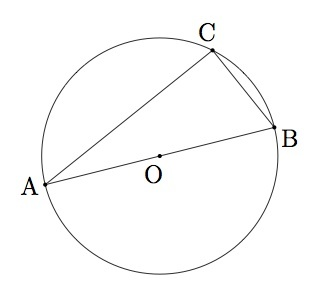# Right triangles inscribed in circles I

Alignments to Content Standards: G-C.A.2

Suppose $\overline{AB}$ is a diameter of a circle and $C$ is a point on the circle different from $A$ and $B$ as in the picture below:1. Show that triangles $COB$ and $COA$ are both isosceles triangles.
2. Use part (a) and the fact that the sum of the angles in triangle $ABC$ is $180$ degrees to show that angle $C$ is a right angle.

## IM Commentary

This task provides a good opportunity to use isosceles triangles and their properties to show an interesting and important result about triangles inscribed in a circle with one side of the triangle a diameter: the fact that these triangles are always right triangles is often referred to as Thales' theorem. It does not have a lot of formal prerequisites, just the knowledge that the sum of the three angles in a triangle is $180$ degrees.

This task can be made substantially more challenging by omitting the auxiliary construction indicated in part (a), or just slightly more difficult by removing some of the explicit guidance from part (b). It can also be further facilitated, if needed, by suggesting for students to study the three angles in triangle $ABC$ and their sum.

As written, the task is suitable for assessment or instruction. If used for instruction, the teacher may wish to make the problem more open ended as indicated in the previous paragraph.

## Solution

1. Below is a picture with segment $\overline{OC}$ added:Since segments $\overline{OB}$, $\overline{OC}$, and $\overline{OA}$ are all radii of the same circle, they are all congruent. Therefore both triangles $COB$ and $COA$ are isosceles triangles.

2. Angles $B$ and $BCO$ are congruent since they are opposite congruent sides of the isosceles triangle $COB$. Similarly angles $A$ and $ACO$ are congruent because they are opposite congruent sides of the triangle $COA$.

The sum of the measures of the three angles in a triangle is $180$ degrees and so $$m(\angle A) + m(\angle B) + m(\angle C) = 180.$$ Using the angle equivalences from the previous paragraph

\begin{align} m(\angle C) &= m(\angle BCO) + m (\angle ACO) \\ & = m(\angle CBO) + m(\angle CAO) \\ & = m(\angle B) + m(\angle A) \end{align}
Substituting this into the previous formula we find $$2m(\angle A) + 2m( \angle B) = 180$$ and so $m(\angle A) + m(\angle B) = 90$. Since the measures of the three angles of triangle $ABC$ add to $180$ this means that $m(\angle C) = 90$ and so triangle $ABC$ is a right triangle as desired.